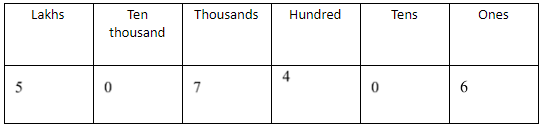QuestionAnswers

# Write the following in numerical form:Five lakh seven thousand four hundred six.Verified
128.7k+ views
Hint:Prepare a place value chart for the numerical form and add the information appropriately in the chart. This will help you to get the answer easily and accurately.

We have to find the numerical form of: Five lakh seven thousand four hundred six.
Before finding the numerical value, we need to understand the place value of the number.
In mathematics, every digit or number has a place value. Place value can be defined as the value represented by a digit in a number on the basis of its position in a certain number.
A place value chart can help us in finding and comparing the place value of the digits in numbers.
Here, we will arrange the numerical form from the right hand side. Such as –
A) Six will be placed in one place.
B) There is no value for tens of places. We will write zero on that place.
C) Seven will be placed in thousand places.
D) There is no value for ten thousand places. We will write zero on that place.
E) At last, five will be placed in lakh places.
The place value chart of the given number is:Hence, the numerical form of Five lakh seven thousand four hundred six is $5,{\text{ }}07,{\text{ }}406$

Note:There are two ways to convert into numerical form: a) Indian system and b) international system.
In the Indian numeral system, the place values of digits go in the sequence of Ones, Tens, Hundreds, Thousands, Ten Thousand, Lakhs, Ten Lakhs, Crores and so on.
In the International number system, the place values of digits go in the sequence of Ones, Tens, Hundreds, Thousands, Ten Thousand, Hundred Thousand, Millions, Ten Million and so on.